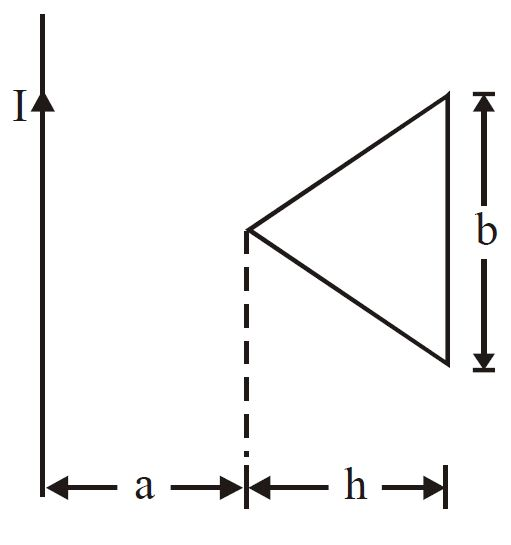# Coefficient of Mutual Induction -2!

A very long straight conductor and isosceles triangular conductor lie in a plane and are separated from each other as shown in the figure. If $a = 10 \text{ cm}$ , $b = 20 \text{ cm}$ and $h = 10 \text{ cm}$, find the coefficient of mutual induction.×